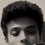# On Ordered relations in the set of complex numbers.

The imaginary numbers were defined to solve equations like
x^2+1=0.

The imaginary unit is defined as
i^2= -1. And any other imaginary number can be written as a product of a real number and the imaginary unit i.

Now, going a bit back to the development of the number system, the following problem was encountered:
x+2=1.

As this equation had no solution in the then existing positive natural number system {1, 2, 3, ...} , a negative unit -1 was defined. Further any equation of the form x+a=b (a>b) could be solved and the solution is the product of the negative unit and the difference of a and b(i.e., a~b).

Ordered relations in complex numbers are undefined because one has no idea about the nature and magnitude and behavior of the imaginary unit i.

Similarly there is no physical or intuitive understanding of the number -1.It is as good as or as bad as the square root of negative one. It was defined to be less than zero, and as the positive number multiplied to -1 increased, we said that the negative number's magnitude decreased. And thus an ordered relation was defined on the set of positive and negative integers. There is no absolute thought or truth regarding the negative numbers. It was simply defined to extend the number system to solve the then unsolvable equations with the then existing number system.

Now, my argument is as follows. Is it wrong to define an ordered relation in complex numbers as follows:

i.b > i.c iff b>c ,

when one can define

-b < -c iff b > c > 0 , where the negative unit -1 is equally non-intuitive and not obvious, and there is no absolute truth regarding the behavior of -1?

That is, to be concise, when we have defined a number such as -1, and assigned ordered relations to it which is not obvious, what is wrong in defining similar ordered relations on imaginary numbers?

This was a question that struck my mind. My argument presumably may be an obviously wrong idea.Note by Samarth M.O.
6 years, 4 months ago

This discussion board is a place to discuss our Daily Challenges and the math and science related to those challenges. Explanations are more than just a solution — they should explain the steps and thinking strategies that you used to obtain the solution. Comments should further the discussion of math and science.

When posting on Brilliant:

• Use the emojis to react to an explanation, whether you're congratulating a job well done , or just really confused .
• Ask specific questions about the challenge or the steps in somebody's explanation. Well-posed questions can add a lot to the discussion, but posting "I don't understand!" doesn't help anyone.
• Try to contribute something new to the discussion, whether it is an extension, generalization or other idea related to the challenge.

MarkdownAppears as
*italics* or _italics_ italics
**bold** or __bold__ bold
- bulleted- list
• bulleted
• list
1. numbered2. list
1. numbered
2. list
Note: you must add a full line of space before and after lists for them to show up correctly
paragraph 1paragraph 2

paragraph 1

paragraph 2

[example link](https://brilliant.org)example link
> This is a quote
This is a quote
    # I indented these lines
# 4 spaces, and now they show
# up as a code block.

print "hello world"
# I indented these lines
# 4 spaces, and now they show
# up as a code block.

print "hello world"
MathAppears as
Remember to wrap math in $$ ... $$ or $ ... $ to ensure proper formatting.
2 \times 3 $2 \times 3$
2^{34} $2^{34}$
a_{i-1} $a_{i-1}$
\frac{2}{3} $\frac{2}{3}$
\sqrt{2} $\sqrt{2}$
\sum_{i=1}^3 $\sum_{i=1}^3$
\sin \theta $\sin \theta$
\boxed{123} $\boxed{123}$

Sort by:

There is this system called lexicographical ordering- that is, a+bi<c+di if and only if a<c or a=c and b<d. This order makes the complex plane totally ordered set. However, you cannot have least upper bound for the subset of the complex plane under this order if your subset does not have a bounded imaginary parts.

- 6 years, 4 months ago

To add more, there is an axiom called axiom of choice which is equivalent to the statement that you can define an order to any arbitrary set. The problem is that the statement only tells us that an order exists, but does not imply properties of the order.

- 6 years, 4 months ago

What would it mean to be positive in the complex plane? Is $i > 0$? You should run into reductio ad absurdum pretty quickly no matter how you answer that question, but I'll let you have the fun of seeing it for yourself.

- 6 years, 4 months ago

Think about how the usual ordering on real numbers is related to addition and multiplication. Would it be possible to extend the ordering to complex numbers in such a way that the properties of multiplication, which you know and love, would continue to hold for all complex numbers?

Staff - 6 years, 4 months ago

No the complex number cannot be an ordered field because i^2=-1.

- 6 years, 4 months ago